# Domain and RangePage 1

#### WATCH ALL SLIDES

Slide 1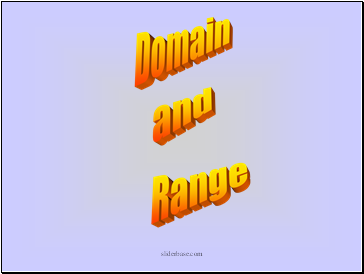## Domain and Range

Slide 2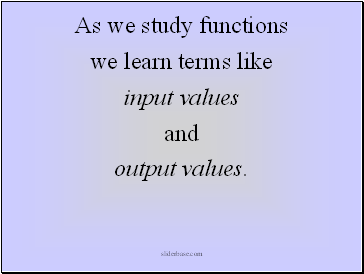As we study functions

we learn terms like

input values

and

output values.

Slide 3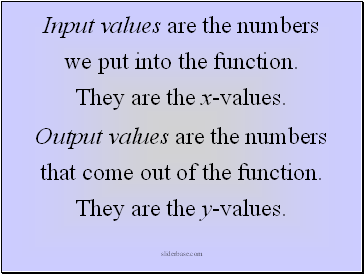Input values are the numbers

we put into the function.

They are the x-values.

Output values are the numbers

that come out of the function.

They are the y-values.

Slide 4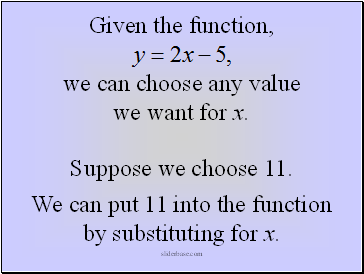Given the function,

we can choose any value we want for x.

Suppose we choose 11.

We can put 11 into the function by substituting for x.

Slide 5Slide 6If we wrote down every number we could put in for x and still have the function make sense,

we would have the set of numbers we call the domain of the function.

Slide 7The domain is the set that contains all the input values for a function.

Slide 8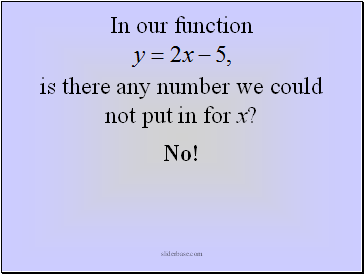In our function

is there any number we could not put in for x?

No!

Slide 9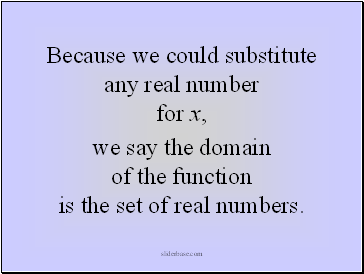Because we could substitute any real number for x,

we say the domain of the function is the set of real numbers.

Slide 10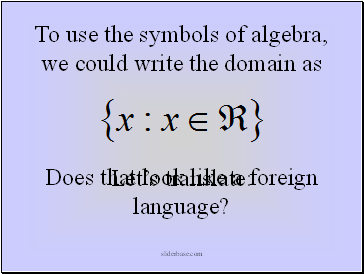To use the symbols of algebra, we could write the domain as

Does that look like a foreign language?

Let’s translate:

Slide 11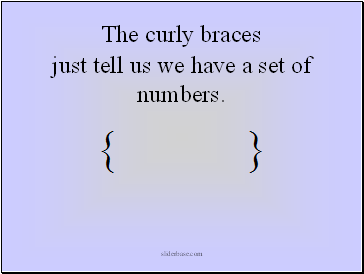The curly braces

just tell us we have a set of numbers.

Slide 12The x reminds us

that our set contains x-values.

Slide 13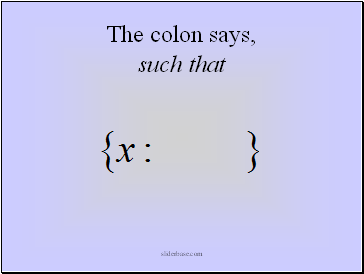The colon says,

such that

Slide 14The symbol that looks like an e

(or a c sticking its tongue out)

says, belongs to . . .

Slide 15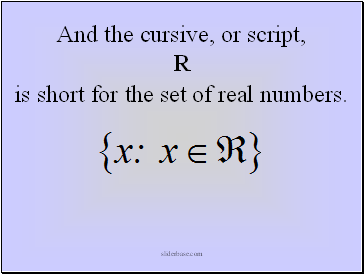And the cursive, or script, R

is short for the set of real numbers.

Slide 16Go to page:
1  2  3